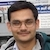# NAEST 2017 Screening Test: Self Made Vernier CalipersBy

A Vernier calipers have two scales (1) main scale and (2) Vernier scale. The least count of the vernier calipers represents its measurement accuracy. The least count is equal to the difference in the lengths of one main scale division and one vernier scale division. This demonstration shows an easy to make vernier calipers.

Question: Choose correct Option(s)

1. The least count of the vernier calipers is 1 cm
2. The least count of the vernier calipers is 0.1 cm
3. The least count of the vernier calipers is 0.01 cm
4. Length of the chalk is within 8.0 cm - 8.2 cm
5. Length of the chalk is within 7.8 cm - 8.0 cm
6. Length of the chalk is more than 8.0 cm
7. Length of the Box is within 4.8 cm - 5.0 cm
8. Length of the Box is within 4.6 cm - 4.8 cm
9. Length of the Box is more than 5.0 cm

Answer: The least count of the Vernier calipers is defined as \begin{align} \text{LC} = 1 \text{MSD} − 1 \text{VSD} \end{align} where MSD is main scale division and VSD is Vernier scale division. In given Vernier scale 1 MSD = 1 cm. Also, 10 VSD = 9 MSD, which gives 1 VSD = (9/10) MSD = 0.9 cm. Thus, \begin{align} \text{LC} = 1\, \text{cm} − 0.9\, \text{cm} = 0.1\, \text{cm} \end{align} In case of chalk, main scale reading (MSR) is 8 and Vernier scale reading (VSR) is 1. Thus, length of the chalk is \begin{align} l& = \text{MSR} + \text{LC}\times \text{VSR} \\ & = 8 + 0.1 \times 1 = 8.1\, \text{cm} \end{align} In case of box, main scale reading (MSR) is 4 and Vernier scale reading (VSR) is 9. Thus, length of the box is \begin{align} l& = \text{MSR} + \text{LC} \times \text{VSR}\\ & = 4 + 0.1 \times 9 = 4.9\, \text{cm}. \end{align} Thus, options (B), (D) and (G) are correct.